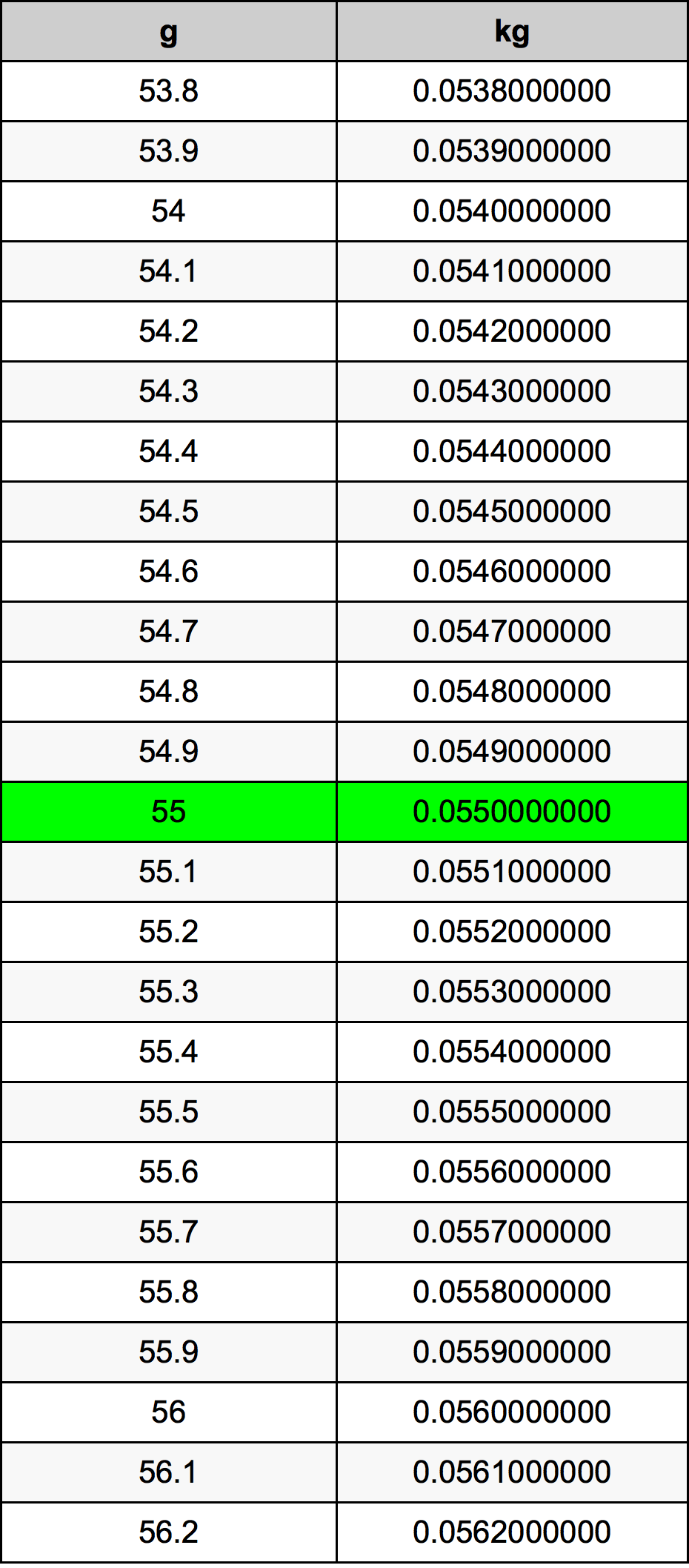Grams To Kilograms

# 55 g to kg55 Grams to Kilograms

g
=
kg

## How to convert 55 grams to kilograms?

 55 g * 0.001 kg = 0.055 kg 1 g
A common question is How many gram in 55 kilogram? And the answer is 55000.0 g in 55 kg. Likewise the question how many kilogram in 55 gram has the answer of 0.055 kg in 55 g.

## How much are 55 grams in kilograms?

55 grams equal 0.055 kilograms (55g = 0.055kg). Converting 55 g to kg is easy. Simply use our calculator above, or apply the formula to change the length 55 g to kg.

## Convert 55 g to common mass

UnitMass
Microgram55000000.0 µg
Milligram55000.0 mg
Gram55.0 g
Ounce1.9400679072 oz
Pound0.1212542442 lbs
Kilogram0.055 kg
Stone0.0086610174 st
US ton6.06271e-05 ton
Tonne5.5e-05 t
Imperial ton5.41314e-05 Long tons

## What is 55 grams in kg?

To convert 55 g to kg multiply the mass in grams by 0.001. The 55 g in kg formula is [kg] = 55 * 0.001. Thus, for 55 grams in kilogram we get 0.055 kg.

## 55 Gram Conversion Table## Alternative spelling

55 g to kg, 55 g in kg, 55 Grams to Kilograms, 55 Grams in Kilograms, 55 Grams to kg, 55 Grams in kg, 55 Grams to Kilogram, 55 Grams in Kilogram, 55 Gram to Kilograms, 55 Gram in Kilograms, 55 g to Kilogram, 55 g in Kilogram, 55 Gram to Kilogram, 55 Gram in Kilogram